# 14-240/Classnotes for Wednesday September 10

During this lecture, we first talked about the properties of the real numbers. Then we applied these properties to the "Field". At the end of the lecture, we learned how to prove basic properties of fields.

## Contents

### The Real Numbers

#### Properties of Real Numbers

The real numbers are a set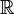$\R$ with two binary operations: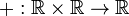$+ : \R \times \R \rightarrow \R$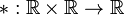$* : \R \times \R \rightarrow \R$


such that the following properties hold.

• R1 :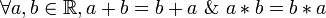$\forall a, b \in \R, a + b = b + a ~\&~ a * b = b * a$ (the commutative law)
• R2 :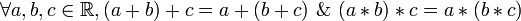$\forall a, b, c \in \R, (a + b) + c = a + (b + c) ~\&~ (a * b) * c = a * (b * c)$ (the associative law)
• R3 :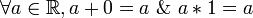$\forall a \in \R, a + 0 = a ~\&~ a * 1 = a$ (existence of units: 0 is known as the

| "additive unit" and 1 as the "multiplicative unit")

• R4 :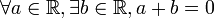$\forall a \in \R, \exists b \in \R, a + b = 0$;

|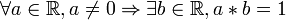$\forall a \in \R, a \ne 0 \Rightarrow \exists b \in \R, a * b = 1$ (existence of inverses)

• R5 :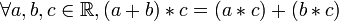$\forall a, b, c \in \R, (a + b) * c = (a * c) + (b * c)$ (the distributive law)

#### Properties That Do Not Follow from R1-R5

There are properties which are true for$\R$, but do not follow from R1 to R5. For example (note that OR in mathematics denotes an "inclusive or"):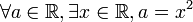$\forall a \in \R, \exists x \in \R, a = x^2$ OR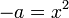$-a = x^2$ (the existence of square roots)


Consider another set that satisfies all the properties R1 to R5. In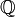$\Q$ (the rational numbers), let us take [/itex]a = 2[/itex]. There is no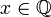$x \in \Q$ such that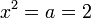$x^2 = a = 2$, so the statement above is not true for the rational numbers!

### Fields

#### Definition

A "field" is a set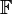$\mathbb{F}$ along with a pair of binary operations: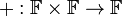$+ : \mathbb{F} \times \mathbb{F} \rightarrow \mathbb{F}$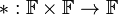$* : \mathbb{F} \times \mathbb{F} \rightarrow \mathbb{F}$


and along with a pair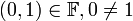$(0, 1) \in \mathbb{F}, 0 \ne 1$, such that the following properties hold.

• F1 :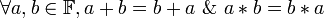$\forall a, b \in \mathbb{F}, a + b = b + a ~\&~ a * b = b * a$ (the commutative law)
• F2 :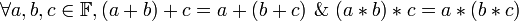$\forall a, b, c \in \mathbb{F}, (a + b) + c = a + (b + c) ~\&~ (a * b) * c = a * (b * c)$ (the associative law)
• F3 :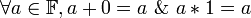$\forall a \in \mathbb{F}, a + 0 = a ~\&~ a * 1 = a$ (existence of units)
• F4 :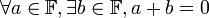$\forall a \in \mathbb{F}, \exists b \in \mathbb{F}, a + b = 0$;

|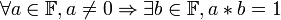$\forall a \in \mathbb{F}, a \ne 0 \Rightarrow \exists b \in \mathbb{F}, a * b = 1$ (existence of inverses)

• F5 :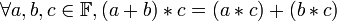$\forall a, b, c \in \mathbb{F}, (a + b) * c = (a * c) + (b * c)$ (the distributive law)

#### Examples

1.$\R$ is a field.
2.$\Q$ (the rational numbers) is a field.
3.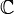$\C$ (the complex numbers) is a field.
4.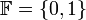$\mathbb{F} = \{0, 1\}$ with operations defined as follows (known as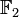$\mathbb{F}_2$ or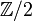$\Z/2$) is a field:
+ 0 1
0 0 1
1 1 0
* 0 1
0 0 0
1 0 1

More generally, for every prime number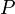$P$,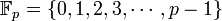$\mathbb{F}_p = \{0, 1, 2, 3, \cdots, p - 1\}$ is a field, with operations defined by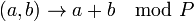$(a, b) \rightarrow a + b \mod P$.

An example: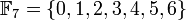$\mathbb{F}_7 = \{0, 1, 2, 3, 4, 5, 6\}$, the operations are like remainders when divided by 7, or "like remainders mod 7". For example,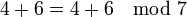$4 + 6 = 4 + 6 \mod 7$ and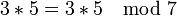$3 * 5 = 3 * 5 \mod 7$.

#### Basic Properties of Fields

Theorem: Let$\mathbb{F}$ be a field, and let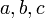$a, b, c$ denote elements of$\mathbb{F}$. Then:

1.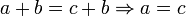$a + b = c + b \Rightarrow a = c$ (cancellation law)
2.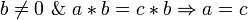$b \ne 0 ~\&~ a * b = c * b \Rightarrow a = c$
     Proof of 1:
1. By F4,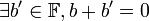$\exists b' \in \mathbb{F}, b + b' = 0$.
We know that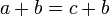$a + b = c + b$;
Therefore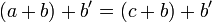$(a + b) + b' = (c + b) + b'$.
2. By F2,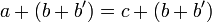$a + (b + b') = c + (b + b')$,
so by the choice of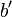$b'$, we know that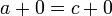$a + 0 = c + 0$.
3. Therefore, by F3,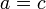$a = c$.
＾_＾

Proof of 2: more or less the same.


3. If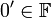$0' \in \mathbb{F}$ is "like 0", then it is 0:

  If$0' \in \mathbb{F}$ satisfies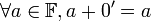$\forall a \in \mathbb{F}, a + 0' = a$, then 0' = 0.


4. If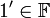$1' \in \mathbb{F}$ is "like 1", then it is 1:

  If$1' \in \mathbb{F}$ satisfies that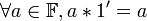$\forall a \in \mathbb{F}, a * 1' = a$, then 1' = 1.

     Proof of 3 :
1. By F3 , 0' = 0' + 0.
2. By F1 , 0' + 0 = 0 + 0'.
3. By assumption on 0', 0' = 0 + 0' = 0.
＾_＾


5.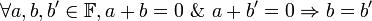$\forall a, b, b' \in \mathbb{F}, a + b = 0 ~\&~ a + b' = 0 \Rightarrow b = b'$:

  In any field "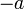$-a$" makes sense because it is unique -- it has an unambiguous meaning.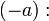$(-a):$ the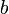$b$ such that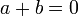$a + b = 0$.


6.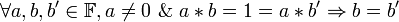$\forall a, b, b' \in \mathbb{F}, a \ne 0 ~\&~ a * b = 1 = a * b' \Rightarrow b = b'$:

  In any field, if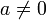$a \ne 0$, "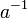$a^{-1}$" makes sense.

     Proof of 5 :
1.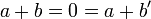$a + b = 0 = a + b'$.
2. By F1,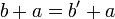$b + a = b'+ a$.
3. By cancellation,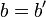$b = b'$.
＾_＾


7.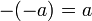$-(-a) = a$ and when$a \ne 0$,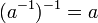$(a^{-1})^{-1} = a$.

     Proof of 7 :
1. By definition,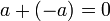$a + (-a) = 0$.          (*)
2. By definition,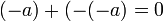$(-a) + (-(-a) = 0$.
3. By (*) and F1,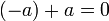$(-a) + a = 0$.
4. By property 5,$-(-a) = a$.
＾_＾